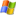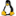Navigation: Home ›  Products ›  Other WaSiM-Helptools ›  Tools for temporal data (time series)

# Tools for processing temporal data (time series)

 idwpTemporal and spatial interpolation of precipitation data, e.g. when merging data of daily resolution with hourly data to create an inner-diurnal course for the daily data by using the temporal information of the neighboring stations in hourly resolution.More » qtospendComputes specific discharges in mm per time step from discharge values in m3/s. quantilComputes the quantiles of the normal distribution, the log-normal distribution (LN2) and the log-normal distribution with lower limit (LN3) using monthly runoff values.More » regrThis is the background version (no interaction) of 'regress'. The program is controlled by a control file (same as for regress, see example in the zip archive). The output files are identical to the regress output, but they are binary, while 'regress' produces ASCII text files. Instead of using regr for external altitude dependend regression, the regression can also be done in WaSiM directly when running the model by using according interpolation methods (10, 11) regrasci+ asciregrConverts 'regr' output files to ASCII format and vice versa. WaSiM is able to use both formats equally. Because of faster access, the binary format may have some advantages when using large files. regressComputing altitude dependend regressions for meteorologic station data (can also be done directly in WaSiM, but this tool has the advantage that the regressions can be shown graphically and thus judged visually). To be able to use this very old program, you should use a DOS emulation like DOS-Box.More » resaggUsed ror aggregation of WaSiM input and output time series in any arbitrary interval length. The output file contains the summed up values for a given number of input intervals. In addition, a total sum will be computed (each value is handled separately per input column). resaggrUsed for aggregation of WaSiM input or output time series. Outputs will contain summed up values for each day, month and year, respectively. An additional total sum is also computed (each value is handled separately per input column) resmeanComputes for each input column of WaSiM input or output time series an average value for each day, month and year, respectively (one file for each temporal output resolution). An additional total sum will be computed. resmeaniComputes for each input column of WaSiM input or output time series an average value for a given number of input intervals. Also, an additional total average will be computed per column. sonneCOmputes interactively sunrise and sunset and draws the diurnal course of the sun as a diagram for each given day of the year and for each given geographical location. DOSbox or another DOS-emulator must be used to use this very old graphic program. sonnerelConverts absolute sunshine durations (e.g. in min per hour) to relative sunshine durations (0..1) spendinvInverts runoff values from mm per time step to time steps per mm (e.g. from mm/h to h/mm) in order to be used for parameter estimation of parameter m for the WaSiM Topmodel version. strlkorrRemoves all negative and (when the sun is under the horizon) small positive direct radiation values from the input time series. all toolsContains all previously listed time series processing tools in a single archive# WaSiM-News

05/15/2020

new release availabe:
WaSiM 10.06.00 -> More »